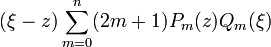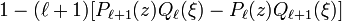User:Tohline/Math/EQ Toroidal06$~(\xi - z)\sum_{m=0}^n (2m+1)P_m(z) Q_m(\xi)$$~=$$~ 1 - (\ell+1)[P_{\ell+1}(z) Q_\ell(\xi) - P_\ell(z)Q_{\ell+1}(\xi)]$ Abramowitz & Stegun (1995), p. 335, eq. (8.9.2)